# A uniform disk turns at 3.7 rev/s around a frictionless spindle. A nonrotating rod, of the same mass as the disk and len... related homework questions

• #### A uniform disk turns at 3.7 rev/s around a frictionless spindle. A nonrotating rod, of the same mass as the disk and len...

A uniform disk turns at 3.7 rev/s around a frictionless spindle. A nonrotating rod, of the same mass as the disk and length equal to the disk's diameter, is dropped onto the freely spinning disk. They then turn together around the spindle with their centers superposed. What is the angular frequency in rev/s of the combination?

• #### A uniform disk turns at 2.7 rev/s around a frictionless central axis. A nonrotating rod, of the same mass as the disk a...

A uniform disk turns at 2.7 rev/s around a frictionless central axis. A nonrotating rod, of the same mass as the disk and length equal to the disk's diameter, is dropped onto the freely spinning disk(Figure 1) . They then turn around the spindle with their centers superposed. What is the angular frequency in rev/s of the combination?

• #### It’s review question, I need this as soon as possible. Thank you 3) For thè diferential equation: (a) The point zo =-1 is an ordinary point. Compute the recursion formula for the coefficients of...It’s review question, I need this as soon as possible. Thank you 3) For thè diferential equation: (a) The point zo =-1 is an ordinary point. Compute the recursion formula for the coefficients of the power series solution centered at zo- -1 and use it to compute the first three nonzero terms of the power series when -1)-s and v(-1)-0. (25 points) (b) Th...

• #### A uniform disk turns at 3.6 rev/s around a frictionless spindle. A non rotating rod, of the same mass as the disk and l...

A uniform disk turns at 3.6 rev/s around a frictionless spindle. A non rotating rod, of the same mass as the disk and length equal to the disk's diameter, is dropped onto the freely spinning disk . They then both turn around the spindle with their centers superposed. What is the angular frequency in rev/s of the combination? please express answer i...

• #### A uniform disk turns at 3.6 rev/s around a frictionless central axis. A nonrotating rod, of the same mass as the dis...

A uniform disk turns at 3.6 rev/s around a frictionless central axis. A nonrotating rod, of the same mass as the disk and length equal to the disk's diameter, is dropped onto the freely spinning disk(Figure 1). They then turn around the spindle with their centers superposed. Figure 1 of 1 Part A What is the angular frequency in rev/s of the combinatio...

• #### Dont copié formé thé book oh ya dont copié formé thé book cause you Oiil inde up being triste soi remembré not toi copié frome thé book oh ya

Dont copié formé thé book oh ya dont copié formé thé book cause you Oiil inde up being triste soi remembré not toi copié frome thé book oh ya!translation in english please!

• #### What is the angular frequency in rev/s of the combination? A uniform disk turns at 2.4 rev/s around a frictionless sp...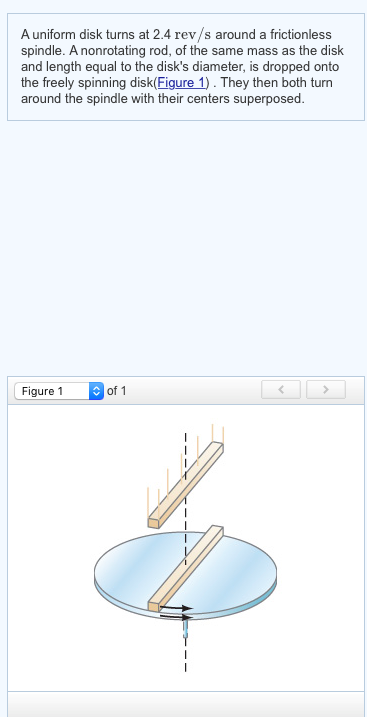What is the angular frequency in rev/s of the combination? A uniform disk turns at 2.4 rev/s around a frictionless spindle. A nonrotating rod, of the same mass as the disk and length equal to the disk's diameter, is dropped onto the freely spinning disk(Figure 1). They then both turn around the spindle with their centers superposed. Figure 1 of 1

• #### Figure 3 Uniform disk Uniform rod 3) Figure 3 illustrates a physical pendulum comprising a uniform disk having mass M and radius R and a rod having the length R and mass M. The disk is pivotally m...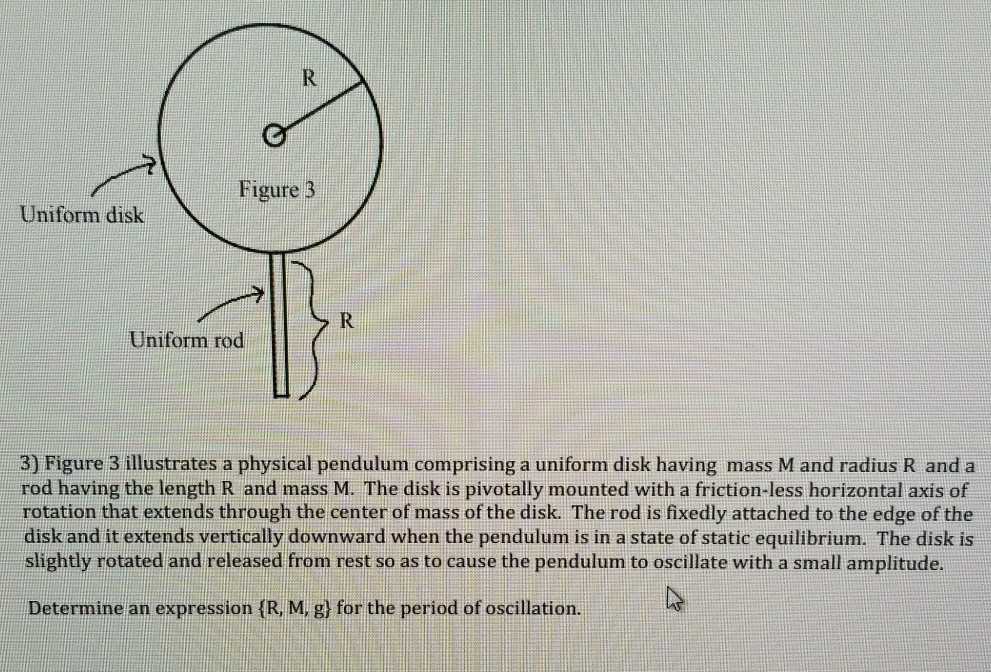Figure 3 Uniform disk Uniform rod 3) Figure 3 illustrates a physical pendulum comprising a uniform disk having mass M and radius R and a rod having the length R and mass M. The disk is pivotally mounted with a friction-less horizontal axis of rotation that extends through the center of mass of the disk. The rod is fixedly attached to the edge of the disk...

• #### A compact disk, which has a diameter of 12.0 cm, speeds up uniformly from zero to 4.40 rev/s in 3.20 s What is the tangential acceleration of a point on the outer rim of the disk at the moment when its angular speed is 3.00 {rev/s}

A compact disk, which has a diameter of 12.0 cm, speeds up uniformly from zero to 4.40 rev/s in 3.20 s What is the tangential acceleration of a point on the outer rim of the disk at the moment when its angular speed is 3.00 {rev/s}?

• #### 6. (8pts) A. The following three boxes show one pair of sister chromatids during spindle formation. What are the identities of Structures A & B? A- B- B. Please draw spindle microtubules to conne...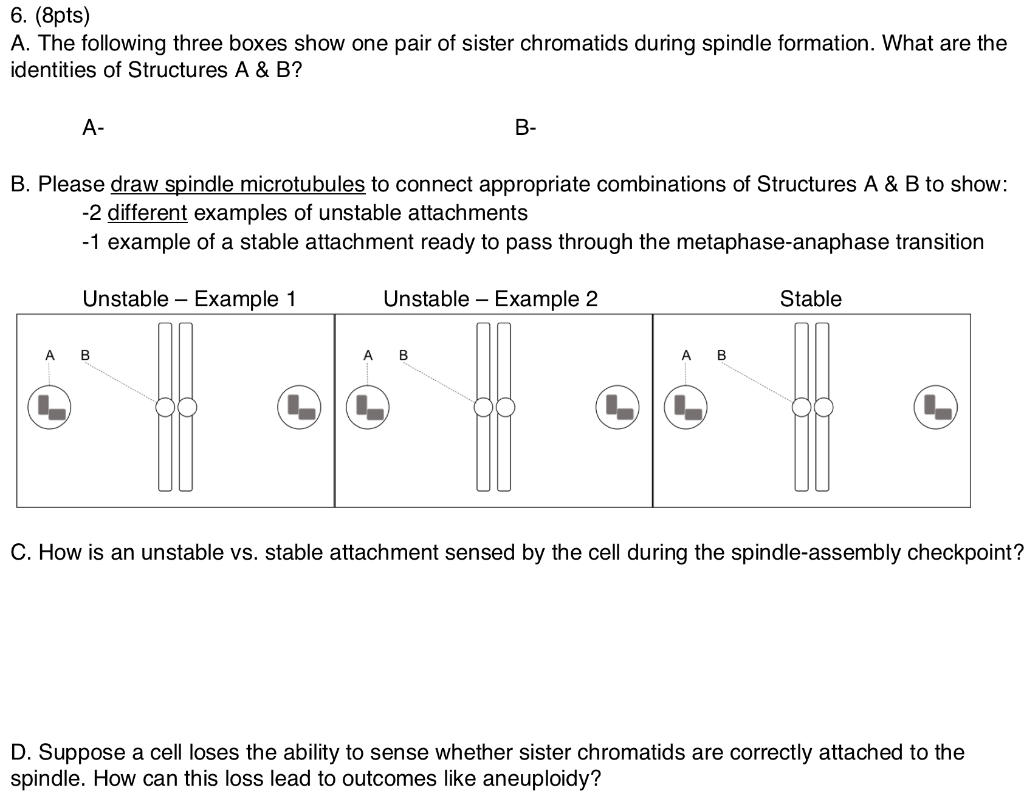6. (8pts) A. The following three boxes show one pair of sister chromatids during spindle formation. What are the identities of Structures A & B? A- B- B. Please draw spindle microtubules to connect appropriate combinations of Structures A & B to show: -2 different examples of unstable attachments 1 example of a stable attachment ready to pass throug...

• #### va ㄧ辽Lai padite.ngenSide.林し ー ess o al len se baliz sentevee and Sinn as Poss va ㄧ辽Lai padite.ngenSide.林し ー ess o al len se baliz sentevee and Sinn as Poss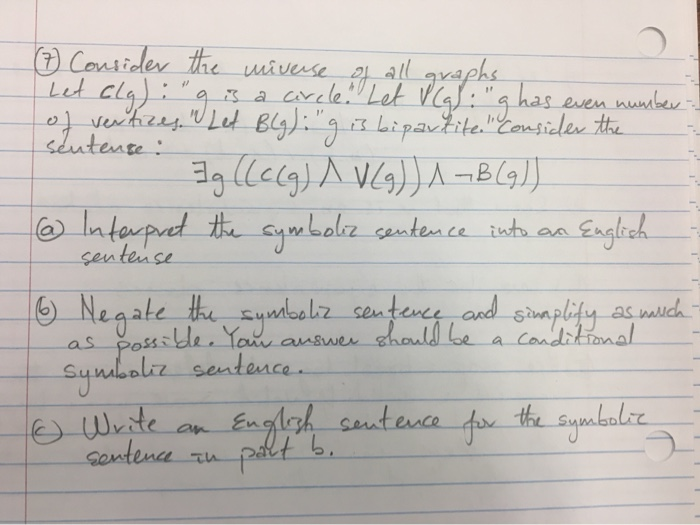va ㄧ辽Lai padite.ngenSide.林し ー ess o al len se baliz sentevee and Sinn as Poss va ㄧ辽Lai padite.ngenSide.林し ー ess o al len se baliz sentevee and Sinn as Poss

• #### def selectionSortK(alist, k): for i in range(0,len(alist) - 1): min = i for j in range(i + 1, len(alist)): if alist[j] < alist[min]: min = j temp = alist[i] alist[i] = alist[min] alist[min] = temp...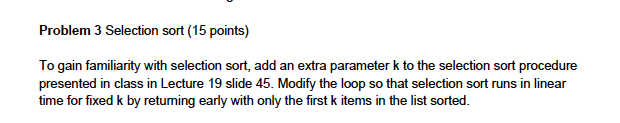def selectionSortK(alist, k): for i in range(0,len(alist) - 1): min = i for j in range(i + 1, len(alist)): if alist[j] < alist[min]: min = j temp = alist[i] alist[i] = alist[min] alist[min] = temp P3: Sanity Test: Is selectionSortK callable? ... ok test_doNothing (__main__.TestProblem3) P3: Does sorting the first k elements with k=0 do nothing? ... ok t...

• #### A potter's wheel is rotating around a vertical axis through its center at a frequency of 2.0 rev/s . The wheel can be considered a uniform disk of mass 4.7 kg and diameter 0.30 m . The potter then throws a 2.8-kg chunk of clay, approximately shaped as a f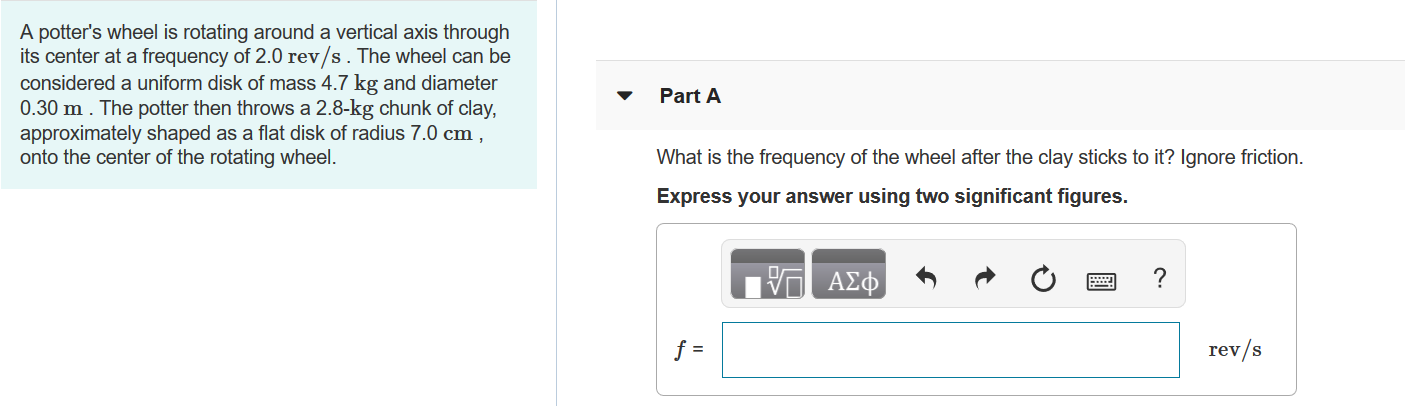A potter's wheel is rotating around a vertical axis through its center at a frequency of 2.0 rev/s . The wheel can be considered a uniform disk of mass 4.7 kg  and diameter 0.30 m . The potter then throws a 2.8-kg chunk of clay, approximately shaped as a flat disk of radius 7.0 cm , onto the center of the rotating wheel.A) What is the frequency of t...

• #### The same as the previous question. The crank AB has a constant clockwise angular velocity of 2000 rpm. The connecting rod is a uniform slender rod whose center of mass is in its geometric cente...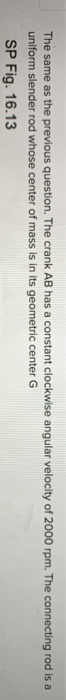The same as the previous question. The crank AB has a constant clockwise angular velocity of 2000 rpm. The connecting rod is a uniform slender rod whose center of mass is in its geometric center G SP Fig. 16.13 に8 in. 40° Check All That Apply ав [-10966, 227 cos 40 -10966.227 sin 40j - 0- -10966. 227 sin 40 + a)BD T, sinß + αΒί cosp -10966. 227 cos 4...

• #### All of t rod of mass B. 2a _x10 kg and length C. 5meters, with two identical uniform hollow spheres, each of mass D. 232x 10 kg a E_ meters, attached at the ends of the rod, as shown below. Not...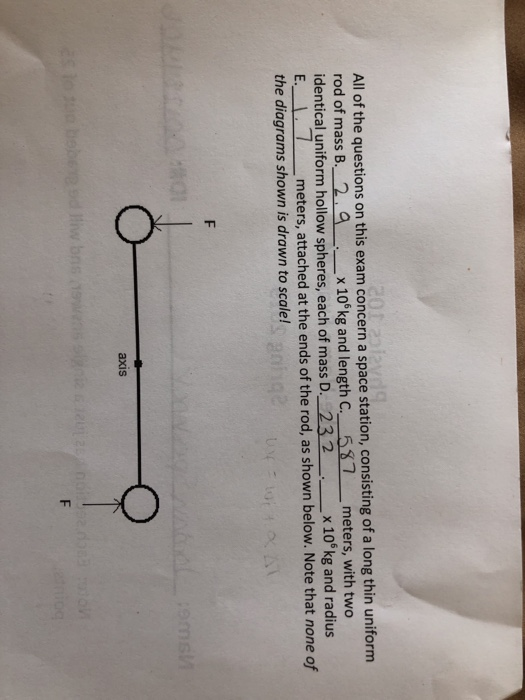All of t rod of mass B. 2a _x10 kg and length C. 5meters, with two identical uniform hollow spheres, each of mass D. 232x 10 kg a E_ meters, attached at the ends of the rod, as shown below. Note that none of the diagrams shown is drawn to scale! he questions on this exam concern a space station, consisting of a long thin uniform nd radius axis axis (e...

• #### M,R a = 0 14. A body consisted of an uniform rod of mass m and length L, and a disk of mass M and radius R can rotate a...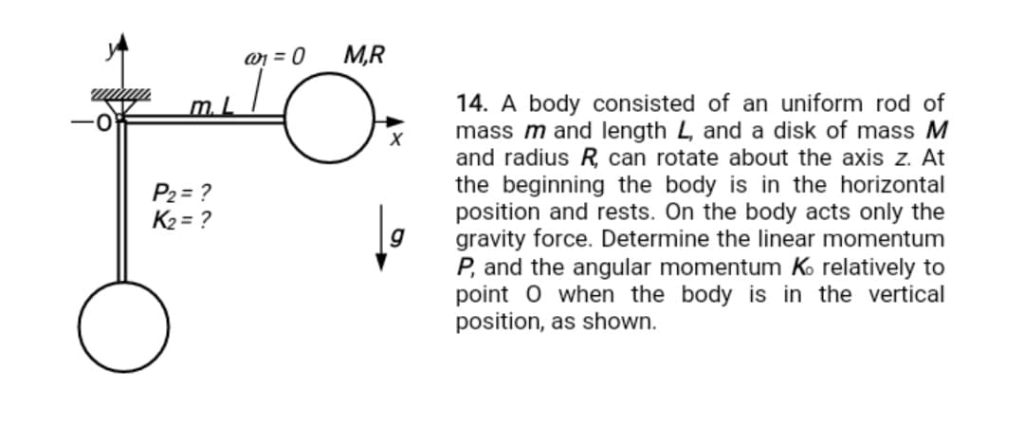M,R a = 0 14. A body consisted of an uniform rod of mass m and length L, and a disk of mass M and radius R can rotate about the axis z. At the beginning the body is in the horizontal position and rests. On the body acts only the gravity force. Determine the linear momentum P, and the angular momentum K relatively to point O when the body is in the vertical...

• #### 44. The system shown in Fig. P7 consists of a slider block of mass m2 and a uniform slender rod of mass m3, length 13, and mass moment of inertia about its center of mass J The slider block is connec...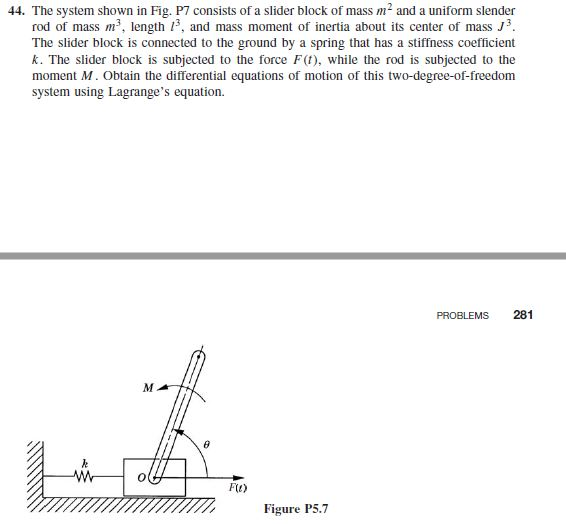44. The system shown in Fig. P7 consists of a slider block of mass m2 and a uniform slender rod of mass m3, length 13, and mass moment of inertia about its center of mass J The slider block is connected to the ground by a spring that has a stiffness coefficient k. The slider block is subjected to the force F(t), while the rod is subjected to the moment M. O...

• #### These questions concern a space station, consisting of a long thin uniform rod of mass 4.3 x 10^6 kg and length 769 meters, with two identical uniform hollow spheres, each of mass 1.7 x 10^6 kg and ra...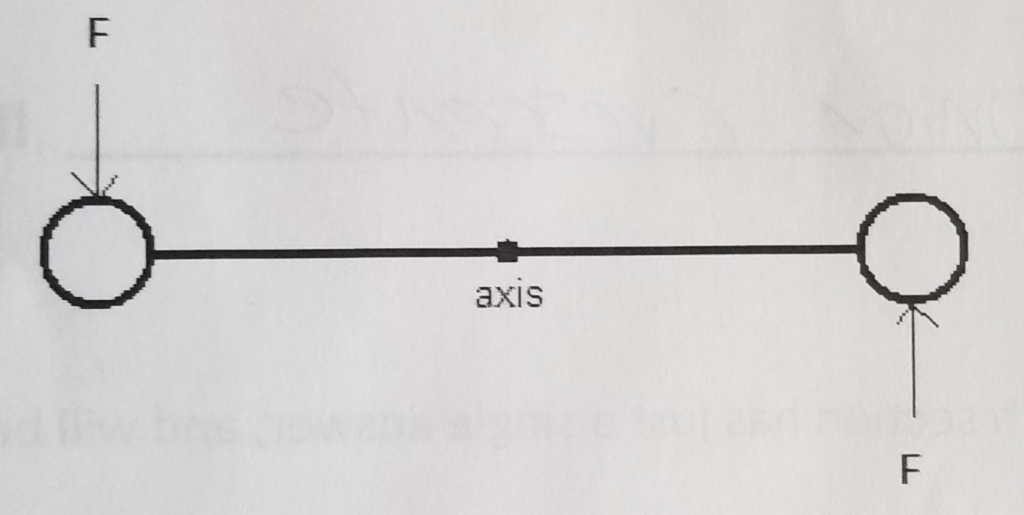These questions concern a space station, consisting of a long thin uniform rod of mass 4.3 x 10^6 kg and length 769 meters, with two identical uniform hollow spheres, each of mass 1.7 x 10^6 kg and radius 218 meters, attached at the ends of the rod, as shown below. Please note that none of the diagrams shown is drawn to scale. A. Suppose that the station st...

• #### All of the questions on this exam concern a space station, consisting of a long thin uniform rod of mass B ax10 kg and length C. 57meters, with two identical uniform hollow spheres, each of mass...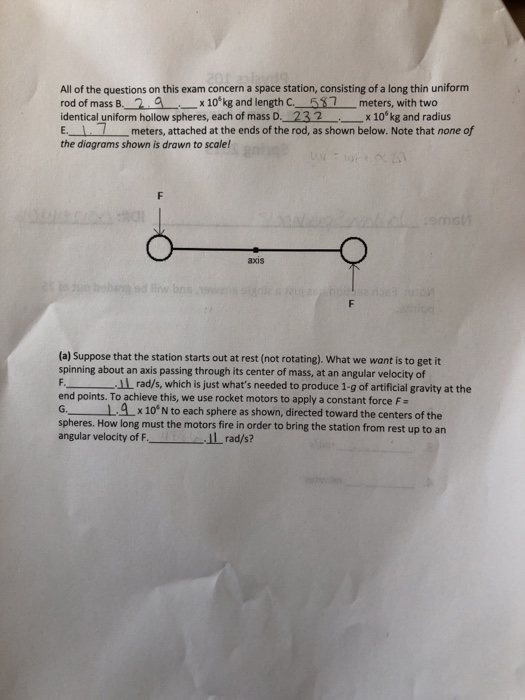All of the questions on this exam concern a space station, consisting of a long thin uniform rod of mass B ax10 kg and length C. 57meters, with two identical uniform hollow spheres, each of mass D._232 x10 kg and radius E. 1 meters, attached at the ends of the rod, as shown below. Note that none of the diagrams shown is drawn to scale! axis (a) Suppose...

• #### All of the questions on this concern a space station, consisting of a long thin uniform rod of mass 4.4 x 106kg and length 171 meters, with two identical uniform hollow spheres, each of mass 1.3 x 106...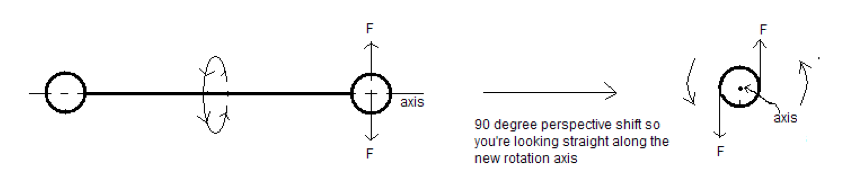All of the questions on this concern a space station, consisting of a long thin uniform rod of mass 4.4 x 106kg and length 171 meters, with two identical uniform hollow spheres, each of mass 1.3 x 106 kg and radius 57 meters, attached at the ends of the rod, as shown below. Note that none of the diagrams shown is drawn to scale! PART D: (d) Let's start...

Need Online Homework Help?